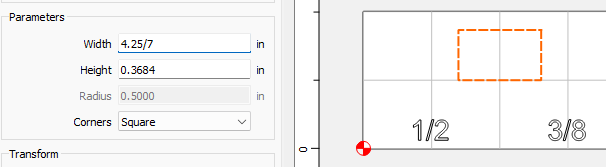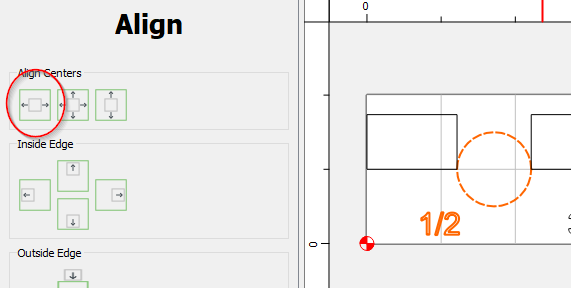# Align objects with even spaces between them

How do I align these circles with even spaces between them, then align the numbers / fractions to the objects?

CC does not have an option to align multiple objects with even spacing, or spacing between objects.
You need to determine with math or geometry the the total amount of space, and divide by the number of spaces. (7 spaces in this example, 5 between the objects, and 2 on the ends)

One way to do this is to line up the objects & measure the remaining space.

Now create another object the size of your space. (4.25 / 7)Copy or Duplicate the object 5 times, and align your object so…

Now align each number with it’s respective circle…
Select the number first, then the circle, then the Left-Right alignment toolRepeat the process for each number / circle pair.
Now Select all of the numbers, And use any of the vertical alignment tools.

You may need to adjust them all together to get even vertical spacing between the circles & bottom edge. Keep them all selected, and manually move the number with the least space, in this case the 1/2.

3 Likes

Another option is to create an array of say the .5" circle. Then drop the smaller circles in the center of the 5 other .5" circles and then delete the .5" circles. For the numbers you select the text first and then the circle and use the align to center then together.

2 Likes

That would align the centers of the circles, with unequal spaces between the circles. He specifically asked for even spaces between the circles.

1 Like